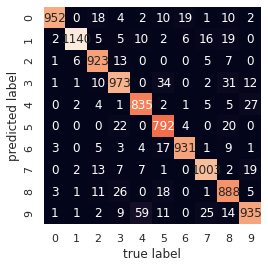Last week on this blog, I presented `AdaOpt` for R, applied to `iris` dataset classification. And the week before that, I introduced `AdaOpt` for Python. `AdaOpt` is a novel probabilistic classifier, based on a mix of multivariable optimization and a nearest neighbors algorithm. More details about the algorithm can be found in this (short) paper. This week, we are going to train `AdaOpt` on the popular MNIST handwritten digits dataset without preprocessing, a.k.a neither convolution nor pooling.

Install `mlsauce`’s `AdaOpt` from the command line (for R, cf. below):

``````!pip install git+https://github.com/thierrymoudiki/mlsauce.git --upgrade
``````

Import the packages that will be necessary for the demo:

``````from time import time
from tqdm import tqdm
import mlsauce as ms
import numpy as np
from sklearn.metrics import classification_report
from sklearn.model_selection import train_test_split
from sklearn.datasets import fetch_openml
``````

Get MNIST handwritten digits data (notice that here, `AdaOpt` is trained on 5000 digits, and evaluated on 10000):

``````Z, t = fetch_openml('mnist_784', version=1, return_X_y=True)

print(Z.shape)
print(t.shape)

t_ = np.asarray(t, dtype=int)

np.random.seed(2395)

train_samples = 5000

X_train, X_test, y_train, y_test = train_test_split(
Z, t_, train_size=train_samples, test_size=10000)
``````

Creation of an `AdaOpt` object:

``````obj = ms.AdaOpt(**{'eta': 0.13913503573317965, 'gamma': 0.1764634904063013,
'k': np.int(1.2154947405849463),
'learning_rate': 0.6161538857826013,
'n_iterations': np.int(245.55517115592275),
'reg_alpha': 0.29915416038957043,
'reg_lambda': 0.163411853029936,
'row_sample': 0.9477046112286693,
'tolerance': 0.05877163298305207})
``````

Adjusting the `AdaOpt` object to the training set:

``````start = time()
obj.fit(X_train, y_train)
print(time()-start)
``````
``````0.7025153636932373
``````

Obtain the accuracy of `AdaOpt` on test set:

``````start = time()
print(obj.score(X_test, y_test))
print(time()-start)
``````
``````0.9372
9.997464656829834
``````

Classification report including additional error metrics:

``````preds = obj.predict(X_test)
print(classification_report(preds, y_test))
``````
``````   precision    recall  f1-score   support

0       0.99      0.94      0.96      1018
1       0.99      0.95      0.97      1205
2       0.93      0.97      0.95       955
3       0.92      0.91      0.91      1064
4       0.91      0.95      0.93       882
5       0.89      0.95      0.92       838
6       0.97      0.96      0.96       974
7       0.95      0.95      0.95      1054
8       0.88      0.93      0.91       953
9       0.93      0.88      0.91      1057

accuracy                           0.94     10000
macro avg       0.94      0.94      0.94     10000
weighted avg       0.94      0.94      0.94     10000
``````

Confusion matrix, true label vs predicted label:

``````import matplotlib.pyplot as plt
import seaborn as sns; sns.set()
from sklearn.metrics import confusion_matrix

mat = confusion_matrix(y_test, preds)
sns.heatmap(mat.T, square=True, annot=True, fmt='d', cbar=False)
plt.xlabel('true label')
plt.ylabel('predicted label');
``````In R, the syntax is quite similar to what we’ve just demonstrated for Python. After having installed `mlsauce`, we’d have:

• For the creation of an `AdaOpt` object:
``````library(mlsauce)

# create AdaOpt object with default parameters

# print object attributes
print(obj\$get_params())
``````
• For fitting the `AdaOpt` object to the training set:
``````# fit AdaOpt to training set
obj\$fit(X_train, y_train)
``````
• For obtaining the accuracy of `AdaOpt` on test set:
``````# obtain accuracy on test set
print(obj\$score(X_test, y_test))
``````

Note: I am currently looking for a gig. You can hire me on Malt or send me an email: thierry dot moudiki at pm dot me. I can do descriptive statistics, data preparation, feature engineering, model calibration, training and validation, and model outputs’ interpretation. I am fluent in Python, R, SQL, Microsoft Excel, Visual Basic (among others) and French. My résumé? Here!